### Image Navigator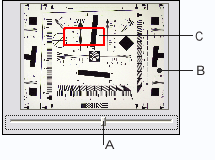A – Zoom slider, drag it to change magnification
B – Thumbnail display of current image
C – Proxy preview area, drag it to move the view of an image

### Region of interest with slanted-edge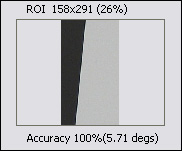ROI 158x291 (26%)” means - ROI size 158x291 and 26% of the distance to the corner.

Accuracy 100%(5.71 degs) means 100% scanned lines of the ROI processed successfully and edge angle equals approximately 5.71 degrees.

### Most important measurements:   CA (chromatic aberration)   MTF50 (50% contrast spatial frequency)   10-90% rise distance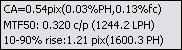CA=0.54pix(0.03%PH, 0.13%fc) means the area chromatic aberration equals 0.54 pixels, 0.03% per image height and 0.13% of the distance from image centre

MTF50: 0.320 c/p (1244.2 LPH) means 50% contrast spatial frequency equals 0.320 cycles per pixel or 1244.2 lines per image height

10-90% rise: 1.21 pix (1600.3 PH) means 10-90% (of maximum energy) rise distance equals 1.21 pixels or 1600.3 rises per image height

### Spatial frequency by contrast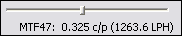MTF47: 0.325 c/p (1263.6 LPH) means 47% contrast spatial frequency equals 0.320 cycles per pixel or 1244.2 lines per image height. Drag the slider to define contrast

### Rise distance by rise range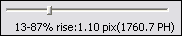13-87% rise: 1.10 pix (1760.7 PH) means 13-87% rise distance equals 1.10 pixels or 1760.7 rises per image height. Drag the slider to define interested range

### MTF level by spatial frequency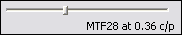MTF28 at 0.36 c/p means spatial frequency 0.36 cycles per pixel corresponds to 28% contrast

### Noise measurements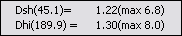Dsh(45.1) = 1.22(max 6.8) means dispersion of high-frequency noise in shadows equals 1.22, average signal level equals 45.1. Maximum quadratic deviation equals 6.8

Dhi(189.1) = 1.30(max 8.0) means dispersion of high-frequency noise in highlights equals 1.30, average signal level equals 189.1. Maximum quadratic deviation equals 8.0

### Channel - R, G, B or Y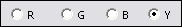Channel selection radio buttons. Current channel can be R, G, B or Y(luminance)

### Gamma setting area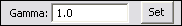To change the gamma, type a value in the edit box and press the Set button

### Selected EXIF data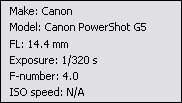Selected EXIF data: Make, Model, Focal length, F-number and ISO speed